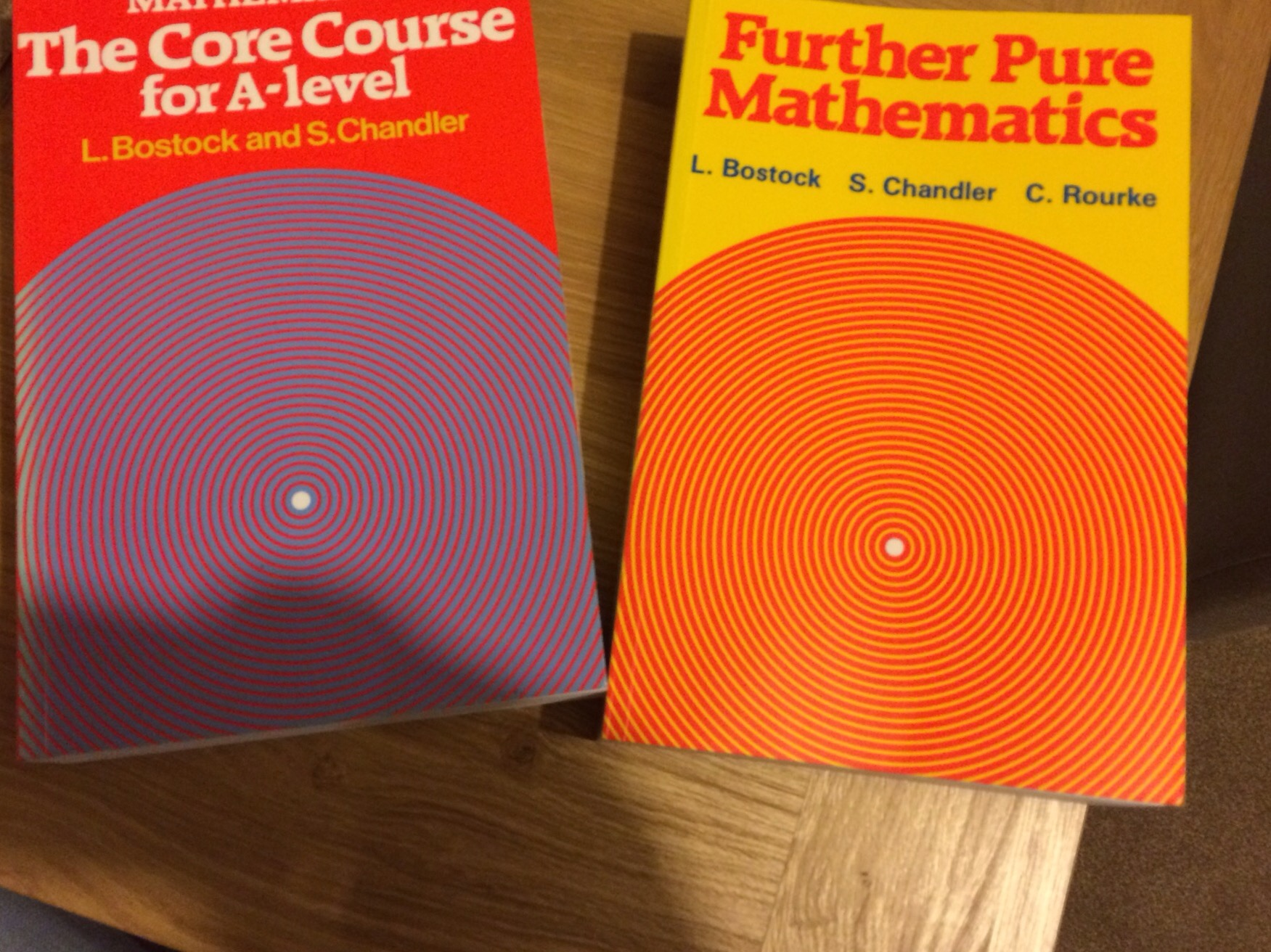Categories

# Classic Maths Books 1 – Bostock and Chandler textbooks

This week I posted the following picture on Twitter:A few people retweeted, favourited and commented how good they were for questions to stretch A Level students so I thought I would write a bit more here.
They were first published in 1982 and are still available from Amazon here and here.
I think I got my copies when I was doing my A Levels and asked my teacher if there were any other books he would recommend that weren’t the Edexcel ones.
I think they are still fantastic books, vastly superior to the Pearson published textbooks, though I think some students may find them a bit dry. They certainly assume more knowledge than the current books and the later questions are generally more involved (or it is less clear on how to start them). There are some really nice examples in the book, such as:

• Find the complex roots of the equation $$2x^2 + 3x + 5 = 0$$. If these roots are $$\alpha$$ and $$\beta$$, confirm the relationships $$\alpha + \beta = – \frac{b}{a}$$ and $$\alpha \beta = \frac{c}{a}$$

All these examples are well explained, and it is easy to follow them line by line.
One other aspect of them that I appreciate are the multiple choice questions at the end of each chapter; these are great for a quick test of understanding and I am using some of them in some QuickKey quizzes that I am trialling this half term.
I may make a blog post about a classic mathematics book a fairly regular thing… gives me an excuse to get some of the older books off my shelf again 🙂